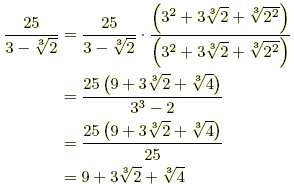# rationalizing the denominator

Rationalizing the Denominator

The process by which a fraction is rewritten so that the denominator contains only rational numbers. A variety of techniques for rationalizing the denominator are demonstrated below.

 Square Roots (a > 0, b > 0, c > 0) Examples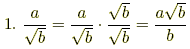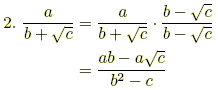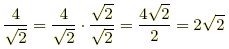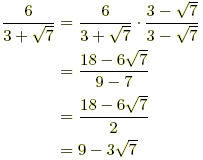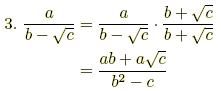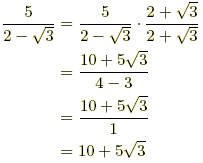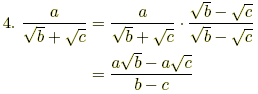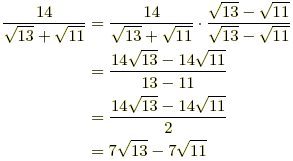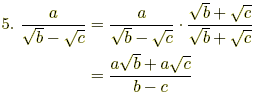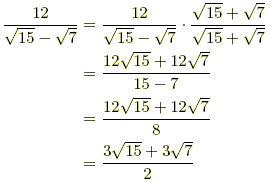nth Roots (a > 0, b > 0, c > 0) Examples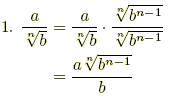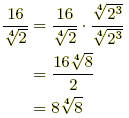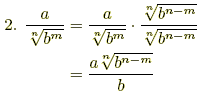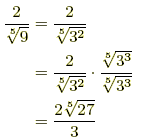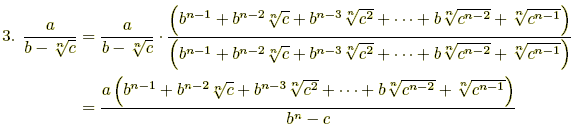Example